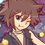# Number Theory (Thailand Math POSN 1st elimination round 2014)

Write a full solution.

1.) Let $a$ be an even number and $b$ an odd number such that $(a,b) = 1$. Find the value of $\left(5^{4a}-1,5^{2b}-1\right)$ using Euclidean algorithm.

2.) (same as last year) Prove that if $p$ and $8p^{2}+1$ are prime numbers, then $8p^{2}+2p+1$ is also prime number.

3.) Find all positive integers $n$ such that

$1^{2557} + 2^{2557} + \dots + n^{2557} + (n+1)^{2557}$

is a composite number.

4.) Let $n \in \mathbb{N}$. Prove that $2^{2^{n+1}} + 2^{2^{n}} + 1$ has at least $n+1$ distinct prime factors.

5.) Let $a,b,c \in \mathbb{N}$ such that $(a,b,c) = 1$ and $\displaystyle \frac{1}{a}+\frac{1}{b} = \frac{1}{c}$. Prove that $a+b$ is a perfect square.

Check out all my notes and stuffs for more problems!

Thailand Math POSN 2013

Thailand Math POSN 2014Note by Samuraiwarm Tsunayoshi
6 years, 9 months ago

This discussion board is a place to discuss our Daily Challenges and the math and science related to those challenges. Explanations are more than just a solution — they should explain the steps and thinking strategies that you used to obtain the solution. Comments should further the discussion of math and science.

When posting on Brilliant:

• Use the emojis to react to an explanation, whether you're congratulating a job well done , or just really confused .
• Ask specific questions about the challenge or the steps in somebody's explanation. Well-posed questions can add a lot to the discussion, but posting "I don't understand!" doesn't help anyone.
• Try to contribute something new to the discussion, whether it is an extension, generalization or other idea related to the challenge.

MarkdownAppears as
*italics* or _italics_ italics
**bold** or __bold__ bold
- bulleted- list
• bulleted
• list
1. numbered2. list
1. numbered
2. list
Note: you must add a full line of space before and after lists for them to show up correctly
paragraph 1paragraph 2

paragraph 1

paragraph 2

[example link](https://brilliant.org)example link
> This is a quote
This is a quote
    # I indented these lines
# 4 spaces, and now they show
# up as a code block.

print "hello world"
# I indented these lines
# 4 spaces, and now they show
# up as a code block.

print "hello world"
MathAppears as
Remember to wrap math in $$ ... $$ or $ ... $ to ensure proper formatting.
2 \times 3 $2 \times 3$
2^{34} $2^{34}$
a_{i-1} $a_{i-1}$
\frac{2}{3} $\frac{2}{3}$
\sqrt{2} $\sqrt{2}$
\sum_{i=1}^3 $\sum_{i=1}^3$
\sin \theta $\sin \theta$
\boxed{123} $\boxed{123}$

Sort by:

1) is obviously 24.2) obviously p=3, which gives a satisfying result(79 is prime).3) is always composite (check divisibility by n or n+1) 5) use SFFT to get (a-c)(b-c)=c^2.But since they are coprime, we get the only sol c^2+c+1,c+1, whose sum is (c+1)^2

- 6 years, 9 months ago

Q.5 Is from RMO-1992(Q.2)

- 6 years, 9 months ago

1)choosing smallest no. of b ( b=1) ...... $5^{2b}$-1 = $5^{2}$-1 = 24

similarly choosing smallest no. of a( a=2) ..... $5^{4a}$-1 = $5^{8}$- 1 (divides 24 as follows:)

$5^{2} \equiv 1 \pmod{24}$

=> $5^{8} \equiv 1 \pmod{24}$

So gcd = 24

3) when no. divided by 2 leaves remainder 0 . no. is composite.

$1 \equiv 1 \pmod{2}$

$1^{1557} \equiv 1 \pmod{2}$...... (1)

$3 \equiv -1 \pmod{2}$

$3^{1557} \equiv -1 \pmod{2}$.......(2)

$1^{1557}+ 3^{1557} \equiv 0 \pmod{2}$

all even to some power will be composite .

So , n= 3,7, 11 ..... should be the nos.

- 6 years, 8 months ago

Someone told me that no.3) every positive integers make the sum composite, but I don't know how to prove that.

- 6 years, 8 months ago